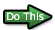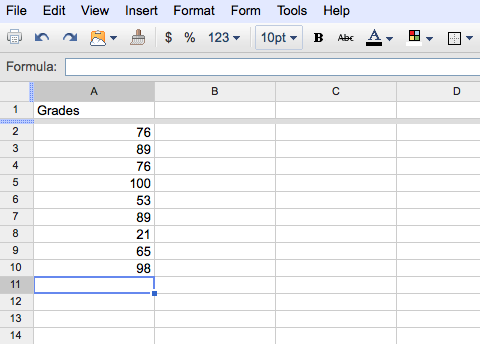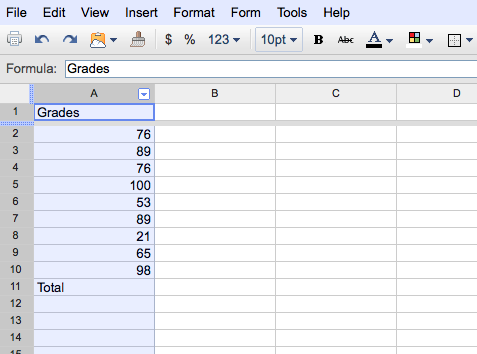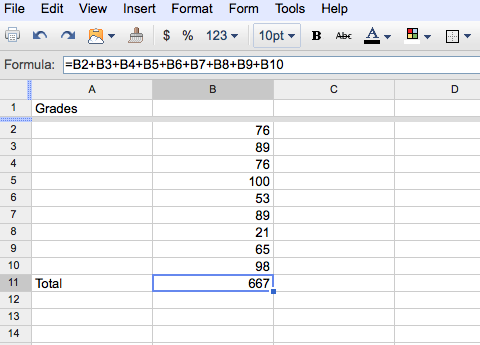# Writing Formulas Using Operators

Operators are what connects the elements of a formula. Some familiar operators are: addition (+), subtraction (-), multiplication (*), and division (/). There is an order of operations when you are evaluating a formula. Formulas are evaluated from left to right, with expressions enclosed in parentheses evaluated first, then exponents, multiplication, division, addition, and subtraction. Spreadsheets has many more operators, but we will work with the operators listed above for now.

Here is an example of how the order of operations works.
If you have the following formula within a cell: =A8/(A9+A4)
The first operation would be the sum of A9 and A4 and then A8 would be divided by that sum.

### Describing Formulas

Spreadsheets allows you to add comments to explain the purpose of an inserted formula. The comments are displayed in the Comment window which can be viewed by choosing Comment from the Insert menu. Let's enter a formula using operators and comment the formula.Open a new workbook window.Starting in cell A1 build the following table:You are going to enter a formula which will calculate the average of these nine grades.Select cell A11 and type in the text: "Total".

It would be best if the total sum of the grades was beneath the last grade. You need to insert a column between column A and column B so you don't have to retype all the numbers.

## Inserting a ColumnHighlight column A by clicking in the column heading.

Observe:Right click on the column A heading and choose Insert 1 Left.

This will insert a new column; column A should be blank now.Select cell B1 and click in the formula bar.Highlight the text: Grades and Cut and Paste the text into cell A1.Cut and Paste the text: Total into cell A11.

Now let's enter the formula for the total sum of the grades.Select cell B11 and enter the following formula:
Remeber to click the Enter/Return key to enter the formula.Your worksheet should look as follows:Select cell A12 and enter the text: Average.Select cell B12 and enter the following formula:orYour worksheet should look as follows:Now let's annotate (comment) the formula in cell B12.

## Annotating Formulas

You can attach notes to cells by using comments. You can view each comment when you rest the pointer over the cell or view all comments at the same time. You can print comments in the same locations where they are displayed on the worksheet or a list at the end of the worksheet. Google Spreadsheets uses the user's name, date, and time in each comment.Select cell B12.Right click and select Insert Comment.

The following Cell Comment box should appear:Within the Comment box, type in the text: Average of nine grades and then click anywhere outside the box.

Observe that there is an orange note indicator in the upper- right corner of the cell. Anytime you move your cursor over the orange note indicator, your comment will appear.

Now that you know how to compute the average using operators, it is time to learn how to write a formula using Spreadsheet's functions.